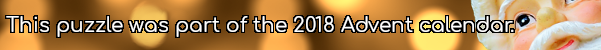mscroggs.co.uk
mscroggs.co.uksubscribe

# Advent calendar 2018

## Archive

Show me a random puzzle
▼ show ▼

## 14 DecemberPut the digits 1 to 9 (using each digit exactly once) in the boxes so that the sums are correct. The sums should be read left to right and top to bottom ignoring the usual order of operations. For example, 4+3×2 is 14, not 10. Today's number is the product of the numbers in the red boxes.
 - + = 10 ÷ + ÷ ÷ + = 3 + - ÷ + × = 33 =7 =3 =3
Tags: numbers, grids
© Matthew Scroggs 2019